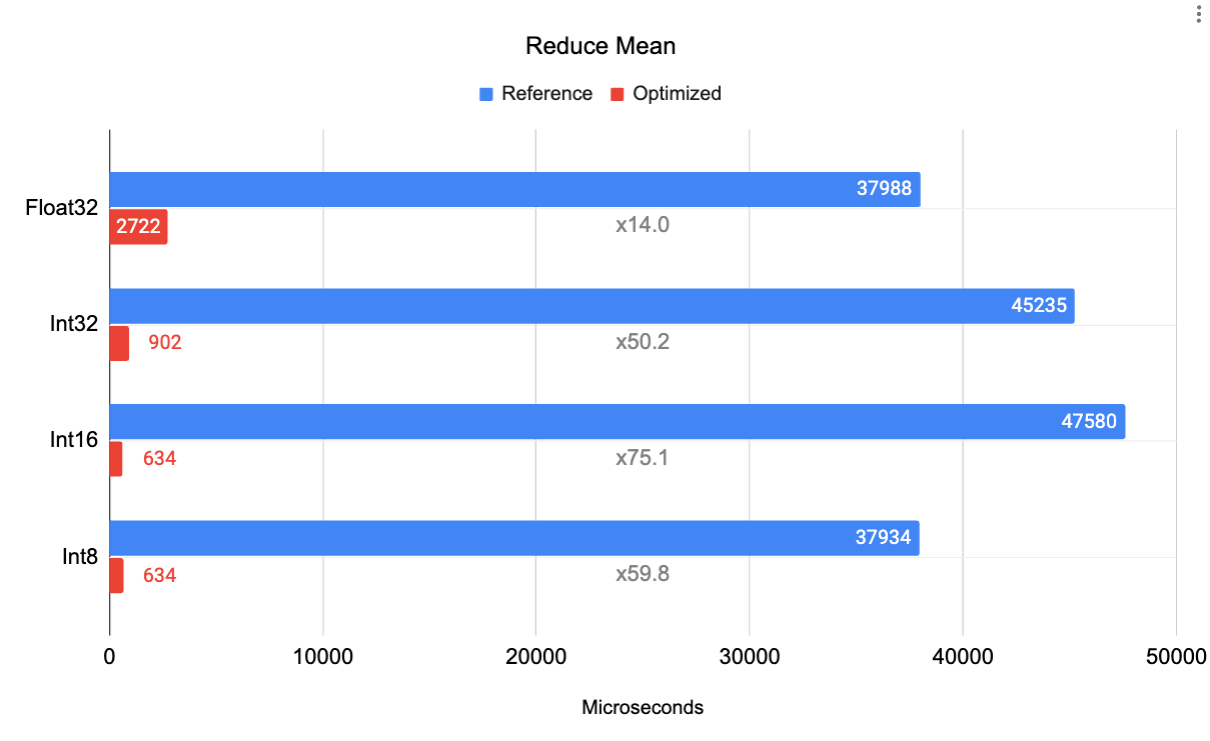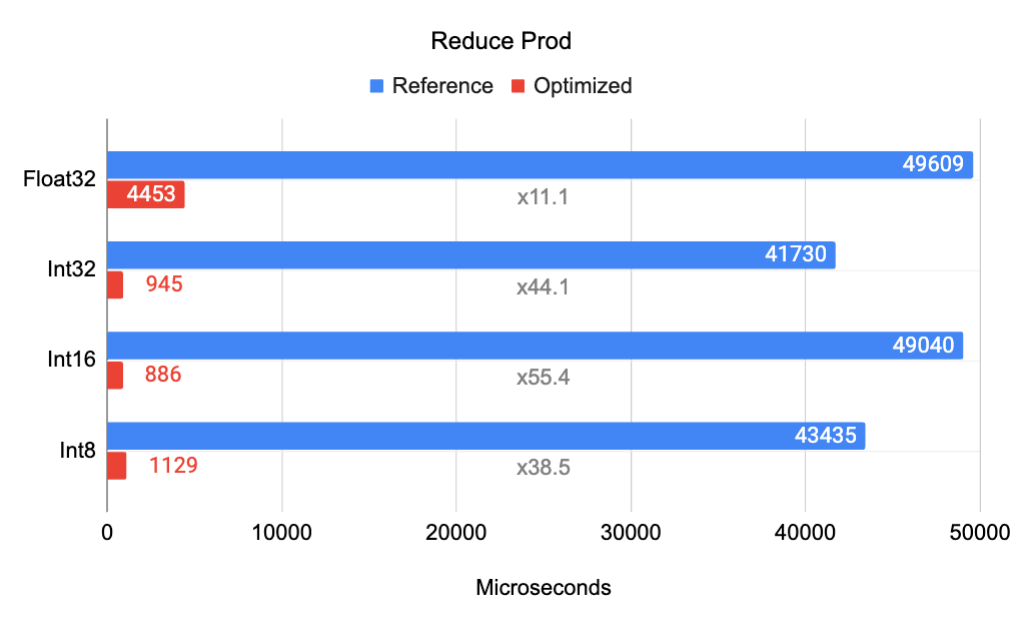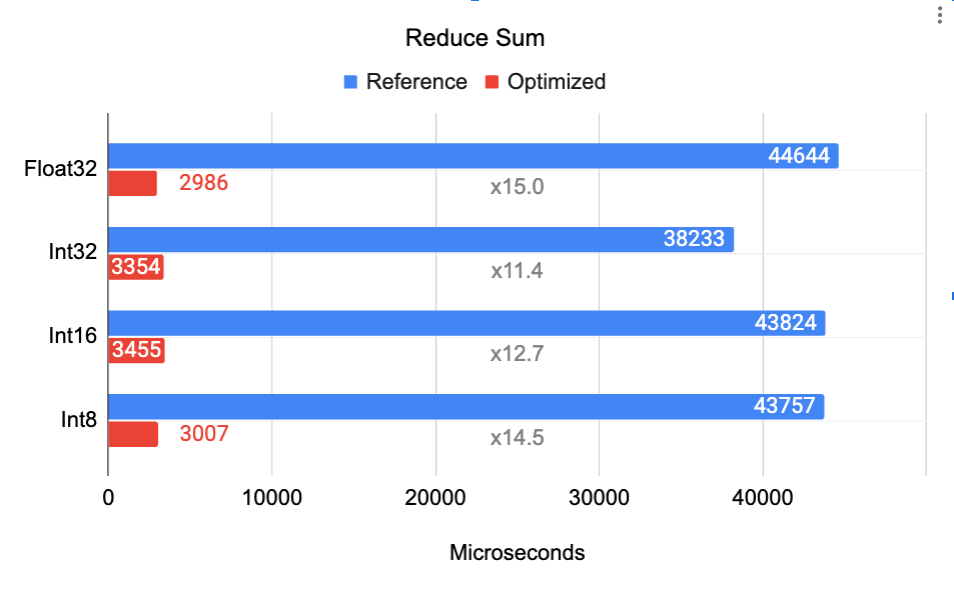# Fast Reduce and Mean in TensorFlow LitePosted by Alan Kelly, Software Engineer

We are happy to share that TensorFlow Lite version 2.10 has optimized Reduce (All, Any, Max, Min, Prod, Sum) and Mean operators. These common operators replace one or more dimensions of a multi-dimensional tensor with a scalar. Sum, Product, Min, Max, Bitwise And, Bitwise Or and Mean variants of reduce are available. Reduce is now fast for all possible inputs.Benchmark for Reduce Mean on Google Pixel 6 Pro Cortex A55 (small core). Input tensor is 4D of shape [32, 256, 5, 128] reduced over axis [1, 3], Output is a 2D tensor of shape [32, 5].Benchmark for Reduce Prod on Google Pixel 6 Pro Cortex A55 (small core). Input tensor is 4D of shape [32, 256, 5, 128] reduced over axis [1, 3], Output is a 2D tensor of shape [32, 5].Benchmark for Reduce Sum on Google Pixel 6 Pro Cortex A55 (small core). Input tensor is 4D of shape [32, 256, 5, 128] reduced over axis [0, 2], Output is a 2D tensor of shape [256, 128].

These speed-ups are available by default using the latest version of TFLite on all architectures.

### How does this work?

To understand how these improvements were made, we need to look at the problem from a different perspective. Let’s take a 3D tensor of shape [3, 2, 5].Let’s reduce this tensor over axes  using Reduce Max. This will give us an output tensor of shape [2, 5] as dimension 0 will be removed. Each element in the output tensor will contain the max of the three elements in the same position along dimension 0. So the first element will be max{0, 10, 20} = 20. This gives us the following output:To simplify things, let’s reshape the original 3D tensor as a 2D tensor of shape [3, 10]. This is the exact same tensor, just visualized differently.Reducing this over dimension 0 by taking the max of each column gives us:Which we then reshape back to its original shape of [2, 5]This demonstrates how simply changing how we visualize the tensor dramatically simplifies the implementation. In this case, dimensions 1 and 2 are adjacent and not being reduced over. This means that we can fold them into one larger dimension of size 2 x 5 = 10, transforming the 3D tensor into a 2D one. We can do the same to adjacent dimensions which are being reduced over.

Let’s take a look at all possible Reduce permutations for the same 3D tensor of shape [3, 2, 5].

Of all 8 permutations, only two 3D permutations remain after we re-visualize the input tensor. For any number of dimensions, there are only two possible reduction permutations: the rows or the columns. All other ones simplify to a lower dimension.

This is the trick to an efficient and simple reduction operator as we no longer need to calculate input and output tensor indices and our memory access patterns are much more cache friendly.

This also allows the compiler to auto-vectorize the integer reductions. The compiler won’t auto-vectorize floats as float addition is not commutative. You can see the code which removes redundant axes here and the reduction code here.

Changing how we visualize tensors is a powerful code simplification and optimization technique which is used by many TensorFlow Lite operators.

### Next steps

We are always working on adding new operators and speeding up existing ones. We’d love to hear about models of yours which have benefited from this work. Get in touch via the TensorFlow Forum. Thanks for reading!Read More